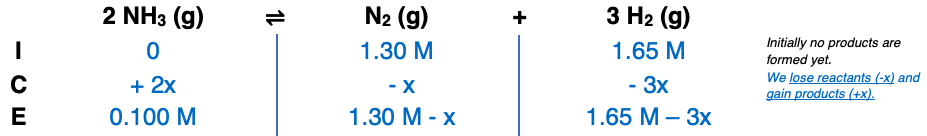# Problem: Consider the formation of ammonia in two experiments. (a) To a 1.00-L container at 727°C, 1.30 mol of N 2 and 1.65 mol of H2 are added. At equilibrium, 0.100 mol of NH3 is present. Calculate the equilibrium concentrations of N 2 and H2, and find Kc for the reaction: 2NH3(g) ⇌ N2(g) + 3H2(g) (b) In a different 1.00-L container at the same temperature, equilibrium is established with 8.34 x 10−2 mol of NH3, 1.50 mol of N2, and 1.25 mol of H2 present. Calculate Kc for the reaction: NH3(g) ⇌ 1/2N2(g) + 3/2H2(g) What is the relationship between the Kc values in parts (a) and (b)? Why aren’t these values the same?

###### FREE Expert Solution

Part A

Step 1

= 1.3 M

= 1.65 M

= 0.1 M

Step 2Step 3

0 + 2x = 0.100 M

x = 0.100 M/2

x = 0.05 M

[N2] = 1.30 M - x

[N2] = 1.30 M - 0.05 M

[N2] = 1.25 M

[H2] = 1.65 M - 3x

[H2] = 1.65 M - 3(0.05M)

[H2] = 1.65 M - 0.15 M

[H2] = 1.50 M

93% (319 ratings)###### Problem Details

Consider the formation of ammonia in two experiments.

(a) To a 1.00-L container at 727°C, 1.30 mol of N 2 and 1.65 mol of H2 are added. At equilibrium, 0.100 mol of NH3 is present. Calculate the equilibrium concentrations of N 2 and H2, and find Kc for the reaction:

2NH3(g) ⇌ N2(g) + 3H2(g)

(b) In a different 1.00-L container at the same temperature, equilibrium is established with 8.34 x 10−2 mol of NH3, 1.50 mol of N2, and 1.25 mol of H2 present. Calculate Kc for the reaction:

NH3(g) ⇌ 1/2N2(g) + 3/2H2(g)

What is the relationship between the Kc values in parts (a) and (b)? Why aren’t these values the same?